[VA] Short & Sweet Math Challenge #25 "San Valentin's Special: Weird Math"
02-20-2021, 11:29 AM
Post: #21J-F GarnierSenior Member Posts: 749 Joined: Dec 2013
RE: [VA] Short & Sweet Math Challenge #25 "San Valentin's Special: Weird Math...
(02-19-2021 11:32 PM)Valentin Albillo Wrote:  Concoction the Second: Weird Sum

The main question is wholly unaddressed: What's so weird about this sum ?. Perhaps a little sleuthing (i.e.: conducting some experiments) would be of help.

I can't explain how this sum converges to 1/2021.
I tried other constant value than 2021, it still worked.

Then I asked myself: are the prime numbers the key for the convergence?
So I calculated the terms, using the suite of natural integers instead of the primes.

1 x 2 x 3 x 4 x 5 x ... (n-1)
--------------------------------
(A+2) x (A+3) x (A+4) x ... (A+n)

Where A is the constant, 2021.

10 A=2021 @ S=0 @ X=1
20 FOR I=1 TO 10
30 X=X*I/(A+I+1) ! next term
40 S=S+X ! sum
50 NEXT I
60 DISP S, 1/S

And guess what? The sum seems to still converge to 1/A

Quite weird, no?

J-F
02-20-2021, 01:37 PM (This post was last modified: 02-20-2021 01:57 PM by C.Ret.)
Post: #22C.RetMember Posts: 218 Joined: Dec 2013
RE: [VA] Short & Sweet Math Challenge #25 "San Valentin's Special: Weird Math...
(02-19-2021 11:32 PM)Valentin Albillo Wrote:
Hi, all:

Concoction the Fourth: Weird Graph

This is mostly unaddressed. In particular no program or description of the operations required to produce the graph have been posted so far and worse, no one has produced and posted the graph itself, i.e.: an actual image of it. This is the kind of functionality for which graphics calculators are intended and I included this part specifically to give HP Prime's or RPL-models' users some opportunity to show off their calculator's worthiness for this challenge.

Also, no attempts to factorize the polynomial have been reported, either successful or unsuccessful. This is the kind of functionality for which CAD is intended. Why don't you give it a try ? Hint: It would help to answer the main question.

Finally, as for the main question proper, What's so weird about this graph?, it's still left unanswered. Apart from its so-described "funny" appearance, there's more to the graph than it seems at first sight. Some sleuthing would surely help.

Thanks and best regards.V.

I spent so much time on these wierd graph that my valentine leave me and she is actualy going out with a former best friend of mine.

The good thing is that I am now full time free of wasting plenty of time on my followed and trusty HP Color Graphing Calculator.

Another good fact is that this polynomial is perfectly even for both arguments x and y this simply greatly the investigation:

$$Po(x,y)=Po(-x,y)=Po(x,-y)=Po(-x,-y)$$

One may investigate it out in the first graph quadrant aka x>=0 and y>=0 only since other quadrants are exact symmetric reflections.I was unable to directly factorize this wierd Polynomial. But by factorizing specific sub-expressions I get information about where to look for roots on specific axis:

$$Po(x,0)=9x^8-100x^6+182x^4-100x^2+9=(x-3)(x-1)^2(x+1)^2(x+3)(3x-1)(3x+1)$$

This clearly indicate that roots on the Ox axis are found at abscissas -3 -1 -1/3 +1 +1/3 +3.
But the squared factors trigger me, I was under alert, there is double root there. Nodes points. Are same time hard to trace on graphics !

$$Po(0,y)=9y^8-4y^6-10y^4-4y^2+9=(y-1)^2(y+1)^2(3y^2-2y+3)(3y^2+2y+3)$$

Fortunately, $$3y^2 ? 2y+3$$ have no real roots , only pure complex zeros. So the only expected zero on the Oy axis are at -1 and +1. Again, double roots alert there .

I was on the way to investigate other specify factorization of $$Po(-3,y) \,Po(-1,y)\,Po(-\frac{1}{3},y)\,\cdots$$ but I realize how powerful is a Graphing Calculator and his Advanced Graphics Application:The position $$Po(x,y)=0$$ are marked by black traces. The graphic windows is from (-3.2,2.4) to (3.2,-2.4). Ticks are 1 unit for both x and y axis. Square zoom 9.
The red color indicate positive values of$$Po(x,y)$$, the darker the highest.
The blue color indicate negative values of $$Po(x,y)$$, the darker the deepest.

The Advanced Graphic App makes a decent job.
But I spent a few hours developing my own way of doing it:The weirdest with this polynomial is to catch the two zeros at (-1,0) and (+1,0) since the polynomial reach zeros there but without any change of sign , all surrounding values stay negative except at the exact zeros points.

Thank a lot for this amazing pulzze. I really spend a good time playing on it !
02-20-2021, 08:47 PM (This post was last modified: 02-20-2021 09:02 PM by robve.)
Post: #23
 robveSenior Member Posts: 360 Joined: Sep 2020
RE: [VA] Short & Sweet Math Challenge #25 "San Valentin's Special: Weird Math...
The weird primes concoction (aka challenge five) depends on the decimal number base. One could argue that a "perfect" property definition should not depend on the number base. The choice of base affects the distribution of base-perfect primes. The prime number theorem sheds some light on the frequency of perfect primes in general.

1. We should observe more primes that meet the definition of base-perfectness when smaller number bases are used than larger number bases.

2. For small primes with k digits such as k=1, k=2, …, k=5 digits there are no perfect primes (in base 10), because primes with k digits are "too close" (as in a Hamming distance kind of way). The prime number theorem tells us that primes are distributed roughly as N/log(N) so that a randomly picked integer not greater than N has a probability of 1/log(N) to be prime. A perfect prime of k digits can be perturbed by its definition to generate 9k distinct composite integers. Roughly, the chance that an integer of k digits is prime is 1/log(10^k)=0.43/k. The chance that the 9k integers are all composite is (1-0.4343/k)^(9k), assuming k is sufficiently large. It turns out that the chance approaches 2% for large k and is half that for small k (though the log constant is somewhat arbitrary). More importantly, there are also far more integers to pick as potential perfect primes for large k. Based on this, it seems reasonable to see perfect primes for large k and there are infinitely many of them.

The first base-perfect primes for base 2 to 19 are (shown is the minimum number of base digits for the perfect prime shown in decimal):

base | digits | first PP   |
2    | 7      | 127        |
3    | 3      | 13         |
4    | 5      | 373        |
5    | 3      | 83         |
6    | 6      | 28151      |
7    | 3      | 223        |
8    | 5      | 6211       |
9    | 4      | 2789       |
10   | 6      | 294001     |
11   | 4      | 3347       |
12   | 7      | 20837899   |
13   | 4      | 4751       |
14   | 6      | 6588721    |
15   | 5      | 484439     |
16   | 5      | 862789     |
17   | 4      | 10513      |
18   | 8      | 2078920243 |
19   | 4      | 10909      |

A very interesting pattern is emerging that has an explanation.

The C "perp" program (note: indent spacing U+00A0):

#include <stdio.h>
#include <stdlib.h>
#include <math.h>

int main(int argc, char **argv)
{
if (argc > 2)
{
long i, j, k;
const long base = atoi(argv); // perfect prime base
const long maxd = atoi(argv); // up to primes of maxd digits
const double lnbase = log(base);
const long max = pow(base, maxd); // primes up to max (exclusive)
char *sieve = (char*)malloc(max); // sieve (0 = composite, 1 = prime)

// init sieve, we should keep only odd values
for (i = 0; i < max; ++i)
sieve[i] = (i & 1);

// seive for primes
for (i = 3; i < max; i += 2)
{
while (i < max && sieve[i] == 0)
++i;
for (j = 2*i; j < max; j += i)
sieve[j] = 0;
}

// sieve for perfect primes
for (i = 3; i < max; i += 2)
{
while (i < max && sieve[i] == 0)
++i;
if (i < max)
{
long m = floor(log(i)/lnbase); // m = number of digits of i
long p = 1; // p = base^j
char h = 1; // h = 1 if i is perfect
// for each jth digit in prime i, from least to most significant
for (j = 0; j <= m; ++j, p *= base)
{
// q = prime i with jth digit zero
long q = i%p + i/p/base*p*base;
// d = jth digit of prime i
long d = i/p%base;
// twiddle jth digit and check for prime
for (k = 0; k < base; ++k)
if (k != d && sieve[q+k*p])
h = 0;
}
if (h)
printf("perfect_%ld %ld\n", base, i);
}
}

free(sieve);
}
else
{
printf("Usage: perp <BASE> <MAXDIGITS>\n");
}
}

- Rob

"I count on old friends" -- HP 71B,Prime|Ti VOY200,Nspire CXII CAS|Casio fx-CG50...|Sharp PC-G850,E500,2500,1500,14xx,13xx,12xx...
02-20-2021, 10:07 PM (This post was last modified: 02-23-2021 01:47 AM by Gerson W. Barbosa.)
Post: #24Gerson W. BarbosaSenior Member Posts: 1,487 Joined: Dec 2013
RE: [VA] Short & Sweet Math Challenge #25 "San Valentin's Special: Weird Math...
(02-19-2021 11:32 PM)Valentin Albillo Wrote:

Concoction the Sixth: Weird Year

Both RPL code and the resulting list of the years have been produced (without comments or explanation), but nearly all the questions have been left unanswered, i.e.:
• What is the "simple but highly remarkable (striking, in fact) numeric property" ?
• (a) How many years will be listed in the output ? ,
• (b) What will be the next predicted potentially catastrophic year after 2020 ?,
• (c) Should we be concerned ?

These were being answered, either explicitly or implicitly, in a post-scriptum to post #17 while you were still writing your post. Sorry for the delay.

(02-19-2021 11:32 PM)Valentin Albillo Wrote:
Finally, programs written in other than RPL would be welcome for variety and to let readers better understand what the code does and how their RPN calcs (say) would deal with the problem.

As you please, on the HP-75C:

10 OPTION BASE 1
15 DIM P(20),Y(100)
20 INPUT L
25 N=CEIL(3*(4*L/LOG(L)^2)^(1/3))
30 FOR I=1 TO 20
40 P(I)=P*P
45 NEXT I
50 C=0
55 FOR I=1 TO N
60 FOR J=1 TO N+1-I
65 S=0
70 FOR K=J TO I+J-1
75 S=S+P(K)
80 NEXT K
85 IF S<=L THEN C=C+1 @ Y(C)=S
90 NEXT J
95 NEXT I
100 FOR I=1 TO C-1
105 FOR J=I TO C
110 IF Y(I)>Y(J) THEN T=Y(I) @ Y(I)=Y(J) @ Y(J)=T
115 NEXT J
120 NEXT I
125 FOR I=1 TO C
130 DISP USING 135 ; Y(I);
135 IMAGE 5d
140 NEXT I
145 DISP
150 END
200 DATA 2,3,5,7,11,13,17,19,23,29,31,37,41,43,47
,53,59,61,67,71

>RUN
?5000
4    9   13   25   34   38   49   74
83   87  121  169  170  195  204  208
289  290  339  361  364  373  377  458
529  579  628  650  653  662  666  819
841  890  940  961  989 1014 1023 1027
1179 1348 1369 1370 1469 1518 1543 1552
1556 1681 1731 1802 1849 2020 2189 2209
2310 2330 2331 2359 2384 2393 2397 2692
2809 2981 3050 3150 3171 3271 3320 3345
3354 3358 3481 3530 3700 3721 4011 4058
4061 4350 4489 4519 4640 4689 4714 4723
4727 4852 4899
>

Best regards,

Gerson.

Edited to fix lack of linguistic precision in the first paragraph
02-21-2021, 04:49 PM
Post: #25
 Nihotte(lma)Member Posts: 74 Joined: Mar 2020
RE: [VA] Short & Sweet Math Challenge #25 "San Valentin's Special: Weird Math...
(02-19-2021 11:32 PM)Valentin Albillo Wrote:
Hi, all:

...

Concoction the First: Weird limit

Point d is still unaddressed:  d. Can you explain the constant component of said [asymptotic] expression ?
...

As stated, Last Chance. Thanks and best regards.V.

I completed my initial result by calculating the rates of increase between the different successive results obtained.
If I consider each f(i) and if I calculate the Rate for i by (f(i) - f(i-1)) / (i - i-1), it will be :
f(1) = 2.71959
f(2) = 4.67827 ---> 1.95868 / (2 - 1) = 1.95868
f(2.021) = 4.71806 ---> 0.03979 / (2.021 - 2) = 1.89476
f(3) = 6.66808 ---> 1.95002 / (3 - 2.021) = 1.99185
f(pi) = 6.95027 ---> 0.28219 / (pi - 3) = 1.99297
f(4) = 8.66601 ---> 1.71574 / (4 - pi) = 1.99843
f(5) = 10.66641 ---> 2.0004 / (5 - 4) = 2.0004
f(5+1/6) = 10.99947 ---> 0.33306 / (5+1/6 - 5) = 1.99836
f(10) = 20.65914 ---> 9.65967 / (10 - (5+1/6)) = 1.99855
f(15) = 30.667 ---> 10.00786 / (15 - 10) = 2.001572
f(20) = 40.66927 ---> 10.00227 / (20 - 15) = 2.000454
and I can see that each time the ratio gives a result really near 2
Each time ? No !
There is one exception with (f(2.021) - f(2)) / (2.021 - 2) = 1.89476
Even, if you evaluate (f(3) - f(2))/ (3 - 2) = 1.98981, the result keeps a sign of this particularity
I can't say if it would possible to notice this lower result in other places that are independent or distant from 2.021 (excluding the portion before i=3, of course), but I can say that is already weird !
02-23-2021, 05:37 PM (This post was last modified: 02-23-2021 05:43 PM by Gerson W. Barbosa.)
Post: #26Gerson W. BarbosaSenior Member Posts: 1,487 Joined: Dec 2013
RE: [VA] Short & Sweet Math Challenge #25 "San Valentin's Special: Weird Math...
#6

Longer, but faster: 17.547 seconds on the HP 50g (previously 29.952 seconds for argument = 5000)

« DUPDUP 4 * SWAP LN SQ / 3 XROOT 3 * CEIL { } 1 DUP2 ROT 5 ROLL
START NEXTPRIME + LASTARG NIP
NEXT DROP SQ DUP SIZE 2 SWAP
FOR i 1 OVER SIZE 1 + i -
FOR j DUP j DUP i + 1 - SUB ∑LIST 4 PICK OVER ≥ { ROT + SWAP } { DROP } IFTE
NEXT
NEXT + SORT REVLIST
REPEAT TAIL
END REVLIST NIP
»

Checksum: # 5E22h
Bytes: 238.5 bytes

This is closer to the HP-75C version, except for the prime list:

« DUPDUP 4 * SWAP LN SQ / 3 XROOT 3 * CEIL { } 1 DUP2 ROT 5 ROLL
START NEXTPRIME + LASTARG NIP
NEXT DROP SQ DUP SIZE 1 SWAP
FOR i 1 OVER SIZE 1 + i -
FOR j DUP j DUP i + 1 - SUB DUP SIZE 1 - NOT { 0 + } IFT ∑LIST 4 PICK OVER ≥ { ROT + SWAP } { DROP } IFTE
NEXT
NEXT ROT DROP2 SORT
»

Checksum: # A346h
Bytes: 226.5 bytes
21.510 seconds

As a comparison, the BASIC program takes about 96 seconds on the HP-75C. Also, the BASIC program is limited to arguments up to 5492, unless of course the prime list is extended.
02-23-2021, 10:28 PM
Post: #27Valentin AlbilloSenior Member Posts: 907 Joined: Feb 2015
RE: [VA] Short & Sweet Math Challenge #25 "San Valentin's Special: Weird Math...

Hi all,

Thank you very much for your overwhelming participation in my S&SMC#25, much, much appreciated. I hope you've enjoyed its two main tasks: sleuthing your way through the challenges, using your brains and your math intuition, then writing efficient code for your favorite HP calcs to let them take away the drudgery. Seeing the many posted solutions and insights, it's quite clear you did well !

Now for my original solutions. Giving the solutions to all six Concoctions at once would make for a tremendously long post which would be most unwieldy to write and to read, so (as I did in some previous S&SMC) I'll give the solutions one Concoction at a time, beginning with the first one, immediately below: first my code and Sleuthing process, then my Results, and finally my extensive Comments. Last but not least, The Hall of Fame !

Note: My HP-71B code may use keywords from the JPC ROM, MATH ROM, HP-IL ROM and STRINGLX LEX file, executed on go71b, while RPN code is for the HP-42S, executed on a DM42.

My original solution for "Concoction the First: Weird Limit"

The Sleuthing

This simple 2-line, 96-byte HP-71B program asks for the sum to exceed, then simulates 10, 100, ..., 100,000 tests and outputs the average counts :

1  DESTROY ALL @ INPUT N @ FOR L=1 TO 5 @ RANDOMIZE 1 @ T=10^L @ M=0 @ FOR I=1 TO T
2  S=0 @ WHILE S<N @ S=S+RND @ M=M+1 @ END WHILE @ NEXT I @ DISP T;M/T @ NEXT L

>RUN
? 1
10      2.7            { error:  0.01828, see below }
100     2.79           { error: -0.07172 }
1000    2.729          { error: -0.01072 }
10000   2.7084         { error:  0.00988 }
100000  2.71959        { error: -0.00131 }

This simpler 24-step, 46-byte RPN program accepts the sum and the number of tests and produces the average count to exceed it:

LBL "SUMRND"  SEED      X<Y?
"Wait..."     LBL 01    GTO 00
AVIEW         CLX       DSE ST Z
STO 01        LBL 00    GTO 01
X<>Y          ISG 00    RCL 00
0            LBL 00    RCL/ 01
STO 00        RAN       CLD
E^X            +        END

1 [ENTER] 1E6 [XEQ] "SUMRND" -> 2.717405     { error: 0.0008768 }
1 [ENTER] 1E7 [XEQ] "SUMRND" -> 2.7180730    { error: 0.0002088 }

Running one or the other (whichever is faster), we get the following results:

N    S =      S = 2      S = 2.021  S = 3      S = Pi     S = 4
---------------------------------------------------------------------
10   2.7        4.8        4.8        6.6        7.1        9.1
100  2.79       4.72       4.75       6.81       7.13       8.85
1E3  2.729      4.701      4.737      6.701      6.978      8.708
1E4  2.7084     4.6751     4.7132     6.6757     6.9571     8.6718
1E5  2.71959    4.67827    4.71806    6.66808    6.95027    8.66601
1E6  2.717405   4.671500   4.712124   6.666287   6.949634   8.664481
1E7  2.7180730  4.6707911  4.7118212     -       6.9500464     -

N    S = 5      S = 5+1/6  S = 10     S = 15     S = 20
------------------------------------------------------------
10   11.2       11.5       20.9       30.6       41.1
100  10.77      11.22      20.66      30.72      40.82
1E3  10.719     11.07      20.623     30.604     40.68
1E4  10.673     11.0006    20.6876    30.7079    40.7027
1E5  10.66641   10.99947   20.65914   30.66700   40.66927
1E6     -          -       20.664967     -          -

The Results

Considering the data obtained above, these are my answers:
• a. What do you think is the average count of generated random numbers for their sum to exceed 1 ? Can you recognize what the exact value would be ?

The limit seems to be ~ 2.7181, which I recognize as the constant e = 2.7182+

• b. What would the average count be for the sum to exceed 2 ? To exceed 2.021 ? To exceed Pi ?

Count(2) = ~ 4.67079, Count(2.021) = ~ 4.71182, Count(Pi) = ~ 6.95004

• c. What do you think is the asymptotic expression for the average count needed to exceed large values of the sum ?

We have Count(5) = ~ 10.666, Count(10) = ~ 20.665, Count(15) = ~ 30.667, Count(20) = ~ 40.669

so the asymptotic expression for large sums S seems to be: Count(S) ~ 2*(S + 1/3)

• d. Can you explain the constant component of said expression ?

The constant in the asymptotic expression seems to be 1/3, and there's a formal explanation but I'll give here an easier, informal one: when the last random number added up results in a sum exceeding the given threshold, the overshoot is also uniformly distributed in [0,1), and the expected value of this overshoot is the expected value of the minimum of two uniform random variables (the random number and the overshoot), which theoretically is 1/3 (i.e.: average of MIN(RND,RND) = 1/3).

If you don't know that piece of theory,this modification of the above HP-71B program will produce it for a given sum by running 10-10,000 tests. It just simulates the process looking at the average overshoot (instead of the average of the count of random numbers generated), and computing the average of the fractional parts (overshoot) of the sum (for the case of integer thresholds), like this:

1  DESTROY ALL @ INPUT N @ FOR L=1 TO 4 @ RANDOMIZE 1 @ T=10^L @ M=0 @ FOR I=1 TO T
2  S=0 @ WHILE S<N @ S=S+RND @ END WHILE @ M=M+FP(S) @ NEXT I @ DISP T;M/T @ NEXT L

>RUN
?           S = 5     S = 10    S = 15
------------------------------------
10      0.356...  0.262...  0.289...
100     0.305...  0.321...  0.276...
1000    0.323...  0.335...  0.333...
10000   0.333...  0.332...  0.333...

The limit average count for the sum of a series of [0,1) uniformly distributed random numbers to exceed 1 is exactly e = 2.71828182845904523536+, the base of the natural logarithms, which is pretty "weird" and can be considered an analog of Buffon's Needle experiment to estimate the value of Pi. Here we don't throw needles on a grid but merrily add up random numbers keeping count and we get e instead.

These are the exact theoretical values for the sum to exceed S:

S  Average Count                               20-decimal value
----------------------------------------------------------------------
1  e                                           2.71828182845904523536
2  e2 - e                                      4.67077427047160499187
3  (2 e3 - 4 e2 + e)/2                         6.66656563955588990415
4  (6 e4 - 18 e3 + 12 e2 - e)/6                8.66660449003269543723
5  (24 e5 - 96 e4 + 108 e3 - 32 e2 + e)/24    10.66666206862241185802

This is the general formula to numerically compute the theoretically exact value ...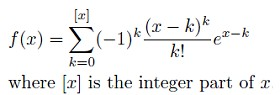... and this is my simple 1-line, 53-byte HP-71B program to instantly compute them given the sum to exceed:

1  DESTROY ALL @ INPUT X @ S=0 @ FOR K=0 TO IP(X) @ S=S+(K-X)^K/FACT(K)*EXP(X-K) @ NEXT K @ DISP S

>RUN
?        1      2.71828182846         2.021      4.71182750642
2      4.67077427047            Pi      6.94950388760
3      6.66656563953         5+1/6      11.0000029914
4      8.66660448999
5      10.6666620697
10      20.6666664745

As the successive terms have alternating signs and go on increasing, the precision for large sums (say > 10) degrades very quickly and we can see that for Sum > 100 the result is but garbage:

100     -2.69702821806E43     { correct value: 200.666666666... }

Implementing the above exact formula in RPN (24 steps, 37 bytes) and running it in the 34-digit DM42 we get the following:

LBL "FSUMRN"   E^X         x
STO 00         LASTX       +
IP             +/-        DSE 01
STO 01         RCL 01     GTO 00
0             Y^X        RCL 00
LBL 00         LASTX      E^X
RCL 00          N!         +
RCL- 01         /         END

A sample run would be:

1 [XEQ] "FSUMRN"   ->    2.71828182846

and assorted results truncated to 20 decimal digits (use [SHOW] to see them in full) would be:

Sum   Average count               Sum     Average count
-------------------------------------------------------------------
1    2.71828182845 904523536    2.021    4.71182750642 763255399
2    4.67077427047 160499187        e    6.10400234136 375415166
3    6.66656563955 588990414       Pi    6.94950388752 954473480
4    8.66660449003 269543722    5+1/6   11.00000299090 420020529
5   10.66666206862 241185801   20+1/6   40.99999999999 999999992
10   20.66666666647 631880061    20.21   41.08666666666 666666663
15   30.66666666666 666034379   21+1/6   43.00000000000 000000000
20   40.66666666666 666666648

which are fully correct to the digits shown. Though the precision attained using the 34-digit DM42 is much greater than using the 12-digit HP-71B program, for large sums it will still quickly degrade. For instance:

50   100.666667031        { only ~ 6 correct decimals, about 9 digits in all }
100   1.12982914443E21     { utter garbage, should be 200.66666666666666666...}

The Hall of Fame

Some of you did bravely tackle this Concoction the First: Weird Limit, namely these four experts:
• robve posted code for the HP Prime, generic BASIC and Python, as well as correct results for questions a and b, gave c a try and left d unanswered. A fine achievement which would be finer had he followed the rules re ony HP models and HP languages, as everyone else did.

• ramon_ea1gth posted RPL code and correctly guessed e as the limit.

• Werner posted RPN code with results identical to robve's post, but correctly guessed the asymptotic expression.

• Nihotte(lma) posted RPN code for the HP-35s and RPL code for the HP48G, correctly guessed the limit to be e and stated that his results match those of robve. He also used a novel (and bold!) thinking-out-of-the-box attempt to guess the asymptotic expression using the HP10BII+ to fit the data to a linear regression, getting correctly the 2*S part, and later trying out yet another approach.

That's all for now, thanks a lot to those who contributed, I really hope you enjoyed it. I'll post my original solutions for "Concoction the Second: Weird Sum" in a couple of days. Stay tuned !Best regards.
V.

All My Articles & other Materials here:  Valentin Albillo's HP Collection

02-24-2021, 09:27 AM
Post: #28
 EdS2Senior Member Posts: 464 Joined: Apr 2014
RE: [VA] Short & Sweet Math Challenge #25 "San Valentin's Special: Weird Math...
Very nice, thanks Valentin! I didn't play, but I enjoy watching the sport.
02-24-2021, 03:42 PM
Post: #29
 robveSenior Member Posts: 360 Joined: Sep 2020
RE: [VA] Short & Sweet Math Challenge #25 "San Valentin's Special: Weird Math...
Valentin, nice result and in-depth investigation.

As a kind suggestion and to offer some respectful constructive feedback: criticizing how we post our results is not helpful. Most of us don't have a lot of time to work on fun stuff. We cannot delay our posts to the end of the week, which is bad for two reasons: 1) it looks like we are just summarizing what other people already posted, and 2) it is not competitive to post our replies very late (because you hinted at some competition for working on these concoctions). If you want more participants and if you want everyone to post in a more organized way then simply do not hint at a competition and produce a Hall Of Fame outcome. It was fun to work on this, but I am not so sure I want to do this again.

Obviously, searching online or looking at other posts totally spoils the fun working on this, at least for me. All results and updates I posted are solely mine! Please note that I mentioned e in my post as the likely result for large trials. I gave no further explanation, as I started thinking about a theoretical result on the expected number of trials for a sum of random variables to exceed a threshold, but that knowledge is no longer at the top of my head, so I let it go and moved on to the other concoctions. Now that the first concoction has ended I verified my gut feeling about this. The result is known to converge to e as the expected value: $$\mathbb{E}[X] = 1 + \sum_{k \geq 1} \Pr[X > k] = 1 + \sum_{k \geq 1} \frac{1}{k!} = e$$ where X is the number of trials you need for the sum to exceed 1. Indeed, a Buffons Needle-like or Monte Carlo approach to estimate e.

Please note that I posted my initial results early and added some new results as I went back to work on the concoctions. I think that most of us approach it that way, because "aha!" moments and inspiration are not constrained to a single day or hour or even a week, arriving with a sort of Gaussian distribution in our heads between your initial post and the deadline, rather than early or late. We also have a day job to take care of first and foremost.

I also thought it would be fine to have my posts combined into one post, deciding to update my initial post with EDIT, which seems reasonable and fair as it indicates what I added or changed. For example, once I went back to work on this I found I misunderstood one formula, corrected my program, and produced the result that you probably looked for so that felt satisfying. In that case it is easy to see that 1/A is the result, which works for any A not only 2021 because 2021 dominates the first primes in the series. Basically, the sum is approximately over terms x^n/(A^n+x^n) and converges to 1/A quickly when 1<x<<A. I don't need to program that further to understand it.

Also, why would you criticize posting extra code like BASIC and Python bad, when posted in addition to HP PRIME code? HP PRIME is not banned, which I had asked. If banned then that should be made more clear and I will no longer participate because I do not own a physical HP-71B or other vintage HP calculator (though I will be on the lookout for a used HP-71B that works when available at a reasonable price.)

To try and run my HP PRIME programs and learn more about the HP PRIME as I go, I spent hours typing them into the HP PRIME on that tiny key pad. I felt that using an virtual calculator on my laptop isn't what you meant these concoctions to be for, since the intent is to actually use the calculators instead of letting them collect dust.

On the subject of calculators collecting dust, the first calculator I used was a HP-45 in the 80s that my Dad owned and cherished. Not sure if he still actively uses it, but he still cannot part with his HP-45!

- Rob

"I count on old friends" -- HP 71B,Prime|Ti VOY200,Nspire CXII CAS|Casio fx-CG50...|Sharp PC-G850,E500,2500,1500,14xx,13xx,12xx...
02-24-2021, 07:01 PM
Post: #30Valentin AlbilloSenior Member Posts: 907 Joined: Feb 2015
RE: [VA] Short & Sweet Math Challenge #25 "San Valentin's Special: Weird Math...
.
Hi, robve:

(02-24-2021 03:42 PM)robve Wrote:  Valentin, nice result and in-depth investigation.

Thanks for your appreciation and most especially for your participation and very comprehensive results.

Never mind, as far as I'm concerned the longer, the better. And this reply of mine isn't small fry, either.

Quote:As a kind suggestion and to offer some respectful constructive feedback: criticizing how we post our results is not helpful.

Could you please give the exact post number of the particular post where I did such criticism ?

Quote:Most of us don't have a lot of time to work on fun stuff. We cannot delay our posts to the end of the week, which is bad for two reasons: 1) it looks like we are just summarizing what other people already posted, and 2) it is not competitive to post our replies very late (because you hinted at some competition for working on these concoctions).

I understand the lack-of-time factor, but as for the "you hinted at some competition for working on these concoctions", that's not so. This is S&SMC #25 and if you have a look at the previous 24 (I know, I know, you don't have the time) you'll see that they've never been posited as "competitions". This one wasn't either.

Quote:If you want more participants and if you want everyone to post in a more organized way then simply do not hint at a competition and produce a Hall Of Fame outcome.

As I've just explained, I don't hint at a competition and never have, that's your own idea, not mine.

And as for the "Hall of Fame", it's a novel idea I had a few days ago (this is the very first time I've included it) and it was intended as a compliment and for showing my appreciation to those people who (like yourself) took the time and effort to post some results, *not* as a score-card or podium or something. Seeing your (over)reaction, perhaps it wasn't such a good idea after all so after posting my 5 remaining solutions (all of which do include their respective "Hall of Fame", I'll drop it for good. Or not.

Quote:It was fun to work on this, but I am not so sure I want to do this again.

It's up to you (", New York, New York."). Anyway, thanks for participating in this one, much appreciated.

Quote:Obviously, searching online or looking at other posts totally spoils the fun working on this, at least for me. All results and updates I posted are solely mine!

That final statement (with exclamation point and all) just reinforces my feeling that you're taking this humble challenge much too seriously. It is and always has been intended for longer than a decade as fun, diversion, never as a competition, that idea is of your own making, not mine. You know, this is not the IMO or Putnam Competition and I won't give you a Fields Medal either so please relax, take it easy !

Quote:Indeed, a Buffons Needle-like or Monte Carlo approach to estimate e.

Yes. And a very simple one, even simpler than Buffon's for Pi. It would be nice to come up with simple stochastic procedures to estimate other ubiquitous math constants.

Quote:Please note that I posted my initial results early and added some new results as I went back to work on the concoctions. I think that most of us approach it that way, because "aha!" moments and inspiration are not constrained to a single day or hour or even a week, [...]. We also have a day job to take care of first and foremost.

I insist, you're taking it too seriously and competitively, and re that "day job first and foremost", nobody is forcing you or expecting you to participate if you can't or won't. Think of this as solving today's paper crosswords or Sudoku: you do it when you have the time and if you feel like it. Same here.

Quote:For example, once I went back to work on this I found I misunderstood one formula, corrected my program, and produced the result that you probably looked for so that felt satisfying.

See ? That's the idea, getting satisfaction. Not stressing over a nonexistent "competitive" edge.

Quote:Also, why would you criticize posting extra code like BASIC and Python bad, when posted in addition to HP PRIME code?

Could you please give the exact post number of the particular post where I did such criticism ?

Quote:On the subject of calculators collecting dust, the first calculator I used was a HP-45 in the 80s that my Dad owned and cherished. Not sure if he still actively uses it, but he still cannot part with his HP-45!

The HP-45 is also the second HP calculator I saw and handled (the first one was a much simpler, cheaper HP-21) and I admired it immensely, utterly state-of-the-art, classy, expensive-looking (and actually !), I so badly wanted one but couldn't afford it as the young adult I was back then. And its hidden-timer functionality was awesome in the extreme (if useless, for lack of a quartz crystal) ! ... Such fond remembrances ...

Thanks for taking the time (be careful, you now: that day job ...) to let me know what you think, Dr. Robert, really much appreciated, wish more people would do that instead of holding everlasting grudges.

In the next days I'll continue to post my original solutions to the remaining 5 Concoctions (which all include their respective "Hall of Fame", sorry) and you may feel relieved to know that afterwards I won't post another S&SMC for a long, long time, if ever, so you'll have no problem in not participating.

Thanks again and best regards.
V.

All My Articles & other Materials here:  Valentin Albillo's HP Collection

02-24-2021, 11:37 PM
Post: #31PeterPMember Posts: 171 Joined: Jul 2015
RE: [VA] Short & Sweet Math Challenge #25 "San Valentin's Special: Weird Math...
thank you Valentin for the wonderful explanations, can't wait to read the ones for the next "reveal".

For what its worth, robve, I have never felt these to be competitions, other than with myself. Most of us struggle with finding the time, though, I totally agree with that. These darn S&SMCs are just so much more fun than the stuff sitting in my inbox :-) (but - at least for me - they can take a lot of time...) I personally learned a lot from your post - I never knew that the Prime can do Python! - so thank you for posting and sharing. This is what makes this place so special, so much to learn from so many incredible people!

Cheers,

PeterP
02-25-2021, 08:24 PM
Post: #32
 robveSenior Member Posts: 360 Joined: Sep 2020
RE: [VA] Short & Sweet Math Challenge #25 "San Valentin's Special: Weird Math...
(02-24-2021 11:37 PM)PeterP Wrote:  For what its worth, robve, I have never felt these to be competitions, other than with myself. Most of us struggle with finding the time, though, I totally agree with that. These darn S&SMCs are just so much more fun than the stuff sitting in my inbox :-) (but - at least for me - they can take a lot of time...) I personally learned a lot from your post - I never knew that the Prime can do Python! - so thank you for posting and sharing. This is what makes this place so special, so much to learn from so many incredible people!
Yes, the challenges are great fun to work on and satisfying to crack. Valentin put a lot of hard work into them. Many kudos. I don't want to sound non-appreciative, which I am not. I certainly don't want to discourage anyone from participating! We are all helping each other to learn something, to improve our knowledge and skills. It is a win-win.

Just giving my feedback on wether or not one could expect comments on posted answers by the OP, which changes the competitive aspect of this IMO. That's all. No drama.

Cheers!

- Rob

"I count on old friends" -- HP 71B,Prime|Ti VOY200,Nspire CXII CAS|Casio fx-CG50...|Sharp PC-G850,E500,2500,1500,14xx,13xx,12xx...
02-25-2021, 09:04 PM (This post was last modified: 02-25-2021 09:22 PM by PeterP.)
Post: #33PeterPMember Posts: 171 Joined: Jul 2015
RE: [VA] Short & Sweet Math Challenge #25 "San Valentin's Special: Weird Math...
Challenge 2, HP41, Sandbox module, unsophisticated, straight forward RPN.

Lbl 'AS
2021
STO 00 ;HP 41 is very slow with numbers
2
+
1/x
STO 01 ; First factor,
STO 02 ; Sum
2
St* 01 ;build second factor
RCL 00
3
STO 11 ;Current Prime
+
ST/ 01
GTO 05 ;calculate sum
LBL 06 ;find next prime
RCL 11 ; current prime
STO L
LBL 00 ;prime finding loop
LastX
2
+
PRIME?
GTO 01
GTO 00
LBL 01
View X ;show current prime
X <> 11 ; Store current prime
X <> 10 ; Put old current prime into last Prime
LBL 02
RCL 10 ; Last Prime
ST* 01
RCL 00
RCL 11
+
ST/ 01 ; update current factor
LBL 05 ; calc sum
RCL 01
ST+ 02
View 02 ; View Current Sum
GTO 06

The sum very quickly converges to 1/2021 or, to be more precise, after some sleuthing using different constants, to 1/A with A being the constant added in the denominator. Some sleuthing shows that its also irrelevant that the sum uses the prime numbers, one could use any sequence of numbers, even a constant 1 (ie sum of 1/(2021+1)^k).

doing a little bit more paper sleuthing on this, one can see that the numerator becomes a O(A^n) and the denominator O(A^(n+1)), meaning the sum trends to 1/A.

Cheers,

PeterP
02-25-2021, 09:29 PM
Post: #34PeterPMember Posts: 171 Joined: Jul 2015
RE: [VA] Short & Sweet Math Challenge #25 "San Valentin's Special: Weird Math...
Challenge 5 - Perfect Primes.

I am clearly not understanding the challenge. Here is what I at first thought it meant:
Quote:A perfect prime is a prime where there is no way you can make it composite by changing one digit (and one digit only) into any other digit.

So the brute force procedure to finding a perfect prime would be
1) Find a prime
2) Take the first digit of the prime
3) change it to all the numbers 0-9 in sequence
4) check each time if the resulting number is a prime number
5) proceed with 2) - 4) for all digits of the prime.
6) if none of the above creates a composite, you have a perfect prime.

Given that all numbers with a sum of the digits divisible by 3 are composite, it seems to me that the above test would always fail, as one could always change one digit up or down enough to make the sum of digits divisible by 3 and hence the number divisible by 3.

Would be great if someone could help me understand what I am missing (which I am sure is blatantly obvious)

Cheers,

PeterP
02-25-2021, 10:40 PM
Post: #35Valentin AlbilloSenior Member Posts: 907 Joined: Feb 2015
RE: [VA] Short & Sweet Math Challenge #25 "San Valentin's Special: Weird Math...
,
Hi, PeterP:

(02-25-2021 09:29 PM)PeterP Wrote:  Challenge 5 - Perfect Primes.

I am clearly not understanding the challenge. Here is what I at first thought it meant:
Quote:A perfect prime is a prime where there is no way you can make it composite by changing one digit (and one digit only) into any other digit.
[...]
Would be great if someone could help me understand what I am missing (which I am sure is blatantly obvious)

With pleassure. You say you thought that it meant:

"A perfect prime is a prime where there is no way you can make it composite by changing one digit (and one digit only) into any other digit."

but actually it's exactly the opposite, it should be "[...] there is no way you can make it prime [...]". Quoting my original definition:

I Wrote:[...] consider a prime number so 'Perfectly Prime' (a PP for short, pronounced "Pepe") that changing any single digit would diminish its primeness by turning it into a composite number. Note: We're talking about base-10 digits here.

I hope that this makes it perfectly clear to you, and thanks for your interest and both recent messages you posted.

V.

All My Articles & other Materials here:  Valentin Albillo's HP Collection

02-25-2021, 11:52 PM
Post: #36Valentin AlbilloSenior Member Posts: 907 Joined: Feb 2015
RE: [VA] Short & Sweet Math Challenge #25 "San Valentin's Special: Weird Math...

Hi all,

As I stated in my earlier post featuring my solution to "Concoction the First: Weird Limit", I'll give the solutions one Concoction at a time, and so here you are, my original solution for "Concoction the Second: Weird Sum", with the same Sections and structure, including The Hall of Fame !

Note: My HP-71B code might use keywords from the JPC ROM, MATH ROM, HP-IL ROM and STRINGLX LEX file, executed on go71b, while RPN code is for the HP-42S, executed on a DM42.

My original solution for "Concoction the Second: Weird Sum"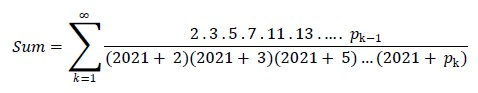The Sleuthing

I'll describe in detail a typical sleuthing procedure to try and answer the above question. First of all, the summation can be expanded as:

Sum = 1/(2021+2) + 2/[(2021+2)*(2021+3)] + 2*3/[(2021+2)*(2021+3)*(2021+5)]
+ 2*3*5/[(2021+2)*(2021+3)*(2021+5)*(2021+7)] + ...

= 1/2023 + 2/4094552 + 6/8295562352 + 30/16823400449856 + ... = 4.94804552195E-4 + ...

and a quick glance convinces me that for 2021 the infinite summation converges very quickly and so I'll use a set of just 10 coefficients (the first 10 primes) to evaluate the sum, which will give more than 12 correct digits. This trivial 4-line, 104-byte HP-71B program will do, let's run it:

1  DESTROY ALL @ INPUT N @ S=0 @ T=1 @ A=1
2  DATA 2,3,5,7,11,13,17,19,23,29
3  FOR I=1 TO 10 @ READ B @ T=T*A/(N+B) @ S=S+T @ A=B @ NEXT I
4  DISP S

>RUN
?   2021 [ENDLINE] -> 4.94804552201E-4

which is the correct 12-digit result. Now, what's this value, can we identify it ?. Well, let's edit line 4 to use the FRAC$keyword to convert the real value to a rational approximation and run the program again: (Note: FRAC$ is a keyword from the JPC ROM, if unavailable you can use my DEC2FRC subprogram to obtain a rational approximation):

4  DISP S;"= ";FRAC$(S) >RUN ? 2021 [ENDLINE] -> 4.94804552201E-4 = 1/2021 so the sum's value is recognized as 1/2021, which is quite unexpected and thus weird. Will this hold for values other than 2021 ?. Let's try some at random: >RUN ? 2028 [ENDLINE] -> 4,93096646943E-4 = 1/2028 ? 1007 [ENDLINE] -> 9.93048659383E-4 = 1/1007 ? -1357 [ENDLINE] -> -7.36919675757E-4 = -1/1357 and yes, it indeed holds for other values ! So, it seems the infinite sum using the set of primes will be equal to the reciprocal 1/N of the given value N, where N = 2021 in the original sum. That using the set of primes we obtain the reciprocal 1/N for other values of N is even weirder but, are we done ? What happens if we use sets other than the prime numbers ? Let's try this by editing the program to use N = 2021 and accept any 10-element set from the user. The edited program looks like this 4-line, 111-byte program: 1 DESTROY ALL @ OPTION BASE 1 @ DIM P(10) 2 S=0 @ T=1 @ A=1 @ N=2021 @ MAT INPUT P @ FOR I=1 TO 10 3 B=P(I) @ T=T*A/(N+B) @ S=S+T @ A=B @ NEXT I 4 DISP S;"= ";FRAC$(S)

Now let's RUN it with assorted sets, namely:

- The set of prime numbers  :  P(1)?  2,3,5,7,11,13,17,19,23,29  ->  4.94804552201E-4 = 1/2021
- The set of natural numbers:  P(1)?  1,2,3,4, 5, 6, 7, 8, 9,10  ->  4.94804552203E-4 = 1/2021
- The set of digits of Pi   :  P(1)?  3,1,4,1, 5, 9, 2, 6, 5, 3  ->  4.94804552202E-4 = 1/2021
- The set of all elements 0 :  P(1)?  0,0,0,0, 0, 0, 0, 0, 0, 0  ->  4.94804552202E-4 = 1/2021
- Any set of random numbers :  P(1)?  RND,RND,RND, {7 more RND}  ->  4.94804552201E-4 = 1/2021

and for the cherry on top, this modified version (edits in bold) will let me demonstrate that the same result is produced when using arbitrary complex sets:

1  DESTROY ALL @ OPTION BASE 1 @ COMPLEX P(10),A,B,S,T
2  S=0 @ T=1 @ A=1 @ N=2021 @ MAT INPUT P @ FOR I=1 TO 10
3  B=P(I) @ T=T*A/(N+B) @ S=S+T @ A=B @ NEXT I
4  DISP S;"= ";FRAC$(REPT(S)) >RUN -> P(1)? (1,3),(0,2),(-3,1),(2.1,1),(1,-2),(0.6,PI),(-3,1),(0,0),(1,5),(10,10) -> (4.94804552201E-4, -1.488..E-18) = 1/2021 The Results Considering all the data obtained above, I can summarize the results as follows: • The value of the original infinite summation is 4.94804552201E-4, identified as 1/2021. What's so weird about this sum ? • For the original summation (N = 2021, set of primes) the sum converges to 1/2021, which is weird. • For any N (real or complex !) such that ABS(N)>1, the sum converges to 1/N, which is weirder. • For any such N, the sum is independent of the set used (even if complex), which is weirdest. The Comments The reason why this infinite summation produces the reciprocal 1/N of a given N (not necessarily 2021, as long as ABS(N)>1) can be better understood by considering the finite sum up to some index K, namely: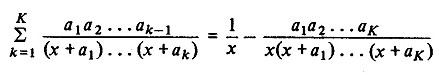where the right-hand side is obtained by expanding the left-hand side sum into its separate terms and noticing that each term is of the form Ak-1 - Ak so that the sum becomes: Sum = (A0 - A1) + (A1 - A2) + (A2 - A3) + ... + (AK-1 - AK) which is a telescoping series: all its terms cancel out except the first and the last, i.e: Sum = A0 - AK, where A0 and AK are as seen in the right-hand side of the above formula. Now, this finite version of the sum does depend on the set of values a1, a2, ..., aK used, as they do clearly appear in the right-hand side of the formula, but when we take it to the limit K -> Inf, then the component A0 = 1/x in the right-hand side remains intact but the other component, which includes all the ak coefficients, tends to 0, and thus the infinite sum becomes independent of the set of ak used and we indeed have that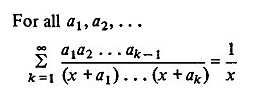The Hall of Fame Again, some of you did bravely tackle this Concoction the Second: Weird Sum, namely these four experts: • robve posted code for the HP Prime and got the correct sum, which he correctly identified, as well as the fact that using other N converges to 1/N. • Werner posted RPN code and also got the correct sum and the correct identification. • J-F Garnier correctly identified the sum and also discovered that other values N converged to 1/N and that using the natural numbers instead of the primes also gave the same sum. • PeterP posted RPN code and correctly identified the sum, and also that using another N converged to 1/N and that using the prime numbers is irrelevant and one could use any sequence of numbers, even a constant 1. That's all for now, as always thanks a lot to those who contributed, I really hope you enjoyed it. I'll post my original solutions for "Concoction the Third: Weird Integral" in a couple of days. Stay tuned !• Note: For any comments not directly related to the math or code here but to ancillary matters such as this or that opinion on the rules or "Halls of Fame" or whatever, please PM me instead of posting them here. Let's keep this thread strictly mathematical and algorithmical in nature. Thanks. Best regards. V. All My Articles & other Materials here: Valentin Albillo's HP Collection 02-26-2021, 07:31 AM Post: #37PeterPMember Posts: 171 Joined: Jul 2015 RE: [VA] Short & Sweet Math Challenge #25 "San Valentin's Special: Weird Math... Thank you Valentin for your kind help, yet to my embarrassment I am still confused. Valentin Wrote:[...] consider a prime number so 'Perfectly Prime' (a PP for short, pronounced "Pepe") that changing any single digit would diminish its primeness by turning it into a composite number. Note: We're talking about base-10 digits here. In another post (apologies for cheating) someone listed the first perfect prime according to their analysis to be 294001. Now, let me change a single digit and diminish its primeness by turning it into a composite number 294003 which is not prime as it is divisible by 3. Now, it is entirely possible that the posted solution of 294001 as a perfect prime is incorrect. But it seems to me that I could change a single digit of any prime number in such a way that the resulting number is divisible by 3. Which would mean there are no perfect primes. Maybe someone can help me understand how 294001 is a perfect prime (or give me any perfect prime and help me understand how and why it is a perfect prime and can’t be made composite by changing any single digit. Thank you so much for your indulgence in helping me understand! Cheers, PeterP 02-26-2021, 07:39 AM Post: #38WernerSenior Member Posts: 747 Joined: Dec 2013 RE: [VA] Short & Sweet Math Challenge #25 "San Valentin's Special: Weird Math... (02-26-2021 07:31 AM)PeterP Wrote: Valentin Wrote:[...] consider a prime number so 'Perfectly Prime' (a PP for short, pronounced "Pepe") that changing any single digit would diminish its primeness by turning it into a composite number. Note: We're talking about base-10 digits here.` Now, it is entirely possible that the posted solution of 294001 as a perfect prime is incorrect. But it seems to me that I could change a single digit of any prime number in such a way that the resulting number is divisible by 3. Which would mean there are no perfect primes. Yes, you can change *a* single digit of any prime to make it divisible by 3. In a perfect prime, however, you could change ANY digit, and it would not be prime anymore. Take 11, for instance. If you change its last digit to 2, it is no longer prime. But if you change it to 3, it IS prime, so 11 is not a perfect prime. The goal is to find primes so that changing any digit would NOT result in another prime. Cheers, Werner 02-26-2021, 10:37 AM Post: #39PeterPMember Posts: 171 Joined: Jul 2015 RE: [VA] Short & Sweet Math Challenge #25 "San Valentin's Special: Weird Math... (02-26-2021 07:39 AM)Werner Wrote: Take 11, for instance. If you change its last digit to 2, it is no longer prime. But if you change it to 3, it IS prime, so 11 is not a perfect prime. The goal is to find primes so that changing any digit would NOT result in another prime. Cheers, Werner LIGHTBULB (i think). In other words, i cannot transform a perfect prime into another prime by changing one digit of it. So if I change any digit of 294001 I will never get another Prime. (Hopefully I got it) Thank you Werner! Cheers, PeterP 02-28-2021, 02:18 AM (This post was last modified: 02-28-2021 11:09 PM by Valentin Albillo.) Post: #40Valentin AlbilloSenior Member Posts: 907 Joined: Feb 2015 RE: [VA] Short & Sweet Math Challenge #25 "San Valentin's Special: Weird Math... Hi all, These are my original solutions for both "Concoction the Third: Weird Integral" and "Concoction the Fourth: Weird Graph", once more with the same Sections and structure, including The Hall of Fame. For my previously posted solutions you can follow these links: "Concoction the First: Weird Limit" and "Concoction the Second: Weird Sum". Note: My HP-71B code might use keywords from the JPC ROM, MATH ROM, HP-IL ROM and STRINGLX LEX file, executed on go71b, while RPN code is for the HP-42S, executed on a DM42. My original solution for "Concoction the Third: Weird Integral"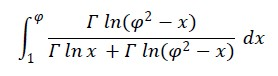What's so weird about this integral? The Sleuthing As before, I'll describe a typical sleuthing procedure to try and answer the above question. First of all, let's compute the integral's value to full 12-digit accuracy using the HP-71B, right from the command line: >DESTROY ALL @ P=(1+SQR(5))/2 [ENDLINE] >INTEGRAL(1,P,0,GAMMA(LN(P^2-IVAR))/(GAMMA(LN(IVAR))+GAMMA(LN(P^2-IVAR)))) [ENDLINE] .309016994376 which is the correct 12-digit result within 1 ulp. In case you didn't recognize it, use my IDENTIFY subprogram (a must for everyone's HP-71B library !) which will identify it as 1/(2*φ) (or an equivalent expression). Now let's create a user-defined function for the f(x) under the integral sign, to experiment a little. This trivial program will do: 1 DESTROY ALL @ P=(1+SQR(5))/2 2 DEF FNF(X)=GAMMA(LN(X)) 3 DISP INTEGRAL(1,P,0,FNF(P^2-IVAR)/(FNF(IVAR)+FNF(P^2-IVAR))) >RUN .309016994376 Now I'll find the value of f(x) and f(φ2-x) at the endpoints of the integration interval: >SFLAG -1 @ FNF(1),FNF(P) @ FNF(P2-1),FNFP(P2-P) @ CFLAG -1 9.99999999999E499 1.84069978531 1.84069978531 9.99999999999E499 and we notice that f(1) ~ Inf, which will do no harm, and that the values at the interval's endpoints for f(x) and f(φ2 - x) are symmetric. Also, from now on we'll use the fact that φ2 = 1 + φ. Experimenting a little, we might compute the integral for other intervals, to see if there's some invariance or anything recognizable, but to no avail. Now, what if we try other functions using the same arguments ? This edited version of the above code (94 bytes) will help us test this idea with assorted user-supplied f(x): 1 DESTROY ALL @ @ P=(1+SQR(5))/2 @ INPUT "f(x)? ";F$
2  DEF FNF(X)=VAL(F\$)
3  DISP INTEGRAL(1,P,0,FNF(P^2-IVAR)/(FNF(IVAR)+FNF(P^2-IVAR)))

>RUN
f(x)?   GAMMA(LN(X))       ->   .309016994376
f(x)?   GAMMA(X)           ->   .309016994375
f(x)?   SQR(LN(X))         ->   .309016994375
f(x)?   SQR(X)             ->   .309016994375
f(x)?   SIN(X)             ->   .309016994375
f(x)?   X^X/COSH(2.021*X)  ->   .309016994375
f(x)?   SIN(SINH(X)+LN(X)) ->   .309016994375
f(x)?   X^3-6*X-2          ->   .309016994375
f(x)?   X                  ->   .309016994375
f(x)?   1                  ->   .309016994375

and it's pretty clear what's happening, so no more sleuthing's needed, let's just go for the results.

The Results

After all the sleuthing above, I can summarize the results as follows:
• The numeric value of the integral is .309016994376 (correct to 12 digits within 1 ulp), identified as 1/(2*φ)   { or also (φ-1)/2 }

• The value of this definite integral is independent of the function being integrated (as long as the same interval and arguments are used, f(x) is continuous and the integral's denominator isn't 0 inside the interval). This is weird !

A key fact is to notice that φ2 equals 1 + φ, so the integral becomes: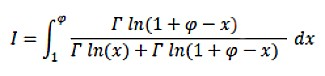and as we also saw above that the values at the endpoints for f(x) and f(φ2 - x) (i.e. f(1 + φ - x)) are symmetric, we perform the change of variable z = 1 + φ - x, dz = -dx, which after trivial algebraic manipulations turns the integral into the form: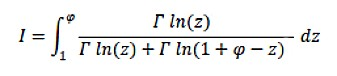and as z is a dummy integration variable, we formally substitute it for x, and the integral becomes now: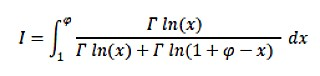which allows for adding this integral to the original one and then we have: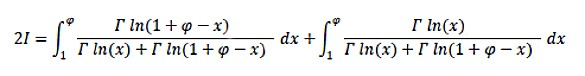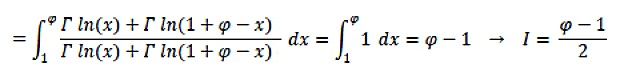and we get the same numerical value we computed earlier, now in symbolic form as (φ-1)/2 = 1/(2*φ) = .309016994375.

Note that this works for any f(x) (subject to the aforementioned limitations) because the expressions in the numerator and denominator in the sum above cancel out and you end up computing the integral of the constant function 1, regardless of the f(x) originally used.

Other intervals [a, b] and arguments (x + u, w - x) are possible as long as w - u = a + b. Here we had a = 1, b = φ, u = 0 and w = φ2 = 1 + φ.

The Hall of Fame

This time the experts which dared to deal with this Concoction the Third: Weird Integral are the following four people:
• J-F Garnier posted RPN code for the HP-32S and said that he successfully computed the numeric value to at least 11 places and had (presumably) deduced what's weird about the integral and its symbolic value as well, though he didn't post any results to avoid spoiling the fun for others.

• robve computed the integral using the HP Prime but alas, the result he posted is wrong. He also posted a BASIC program for the SHARP PC-1350 which produced the same wrong result. Anyway, thanks for trying ...

• Werner posted RPN code for the HP-42S to be used with the built-in integration functionality but he didn't post any results, presumably to avoid spoiling the fun, as J-F did. In a subsequent message he explained his sleuthing and gave both the answer to the "What's weird" question as well as the correct symbolic value.

• Nihotte(lma) posted an RPN program for the HP35s and correctly computed the integral's numeric value, which he also identified symbolically as well.

My original solution for "Concoction the Fourth: Weird Graph"

"Consider the following polynomial in two real variables x, y:

P(x, y) = 9 x8 + 9 y8 + 36 x2 y6 + 54 x4 y4 + 36 x6 y2 - 100 x6 - 4 y6 - 108 x2 y4 - 204 x4 y2 + 182 x4 - 10 y4 - 84 x2 y2 - 100 x2 - 4 y2 + 9

Plot the graph of P(x, y) = 0.  What's so weird about the graph ?"

The Sleuthing

In this case, the first thing to do is, well, to plot the graph of P(x, y) = 0. As I stated in my OP, I won't post code or manual operations as I don't own any graphing calculators but I'll give the resultant graphic you should get, which comes out like this (Note: ignore the line segments and labels for now, they're explained in the Comments below):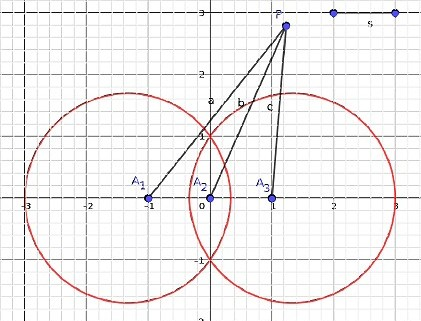And we can see that the graph is perfectly symmetrical respective of both X,Y axes and seems to consist of two intersecting circles (in red, the "crossed eyes") and two isolated points ("the pupils"), (-1, 0) and (1, 0), labeled as A1 and A3, respectively.

The graph in itself is funny-looking (thus weird) but is that all there's to it ? Let's explore the isolated points by looking at the intersections of P(x, y) = 0 with the X axis, i.e. by looking at the roots of P(x, 0) = 0, which we obtain by factorizing that polynomial:

P(x, 0) = 9 x8 - 100 x6 + 182 x4 - 100 x2 + 9 = 0, which factorizes as (x + 3)(3 x + 1)(x + 1)2(x - 3)(3 x - 1)(x - 1)2 = 0,

and we see that the graph intersects the X axis at the points (-3,0), (-1/3, 0), (3, 0), (1/3, 0) and also (-1, 0), (1, 0) which both turn out to be double roots, so each of them is a double isolated point of the graph. Their isolation means that there are no other graph points in their vicinity, which makes them doubly weird, thus weirder.

Are we done ? Not yet. The "crossed eyes" part of the graph certainly seems to consist of two perfect circles at first sight but, is that so ? Are they true circles ?. The question can be answered by factorizing P(x, y) and checking whether there are two factors which can be identified as the respective circles' equations, e.g.:

Q(x, y) = 3 x4 + 3 y4 + 6 x2 y2 - 6 x2 - 10y2 + 3    factorizes as   3 (x2 + y2 + 2√3/3 y - 1) (x2 + y2 - 2√3/3 y - 1),

and both 2nd-degree polynomial factors are equations of circles so the graph of Q(x, y) = 0 is the union of two true circles. Regrettably, our P(x, y) does not factorize that way so let's zoom in the first quadrant (the others are symmetrical) at suitably high magnification, and we get this view, where the graph of P(x, y) appears in red and a superimposed true circle appears in black: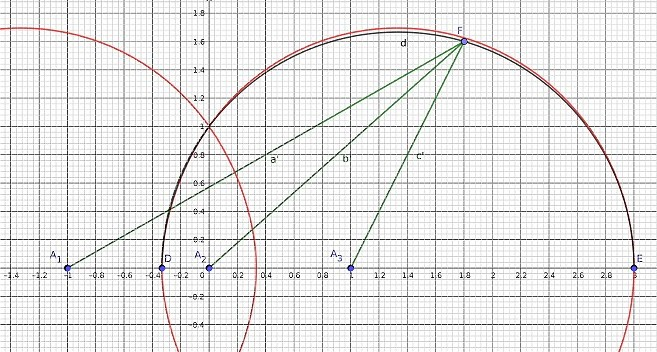As you can see, they don't exactly match, close but no cigar !   This means that the "crossed eyes" ara not true circles in fact, and that is most unexpected and thus weirdest. Enough sleuthing, time for the results.

The Results

Once the sleuthing is over, I can summarize the results as follows:
• The graph consists of what seems to be two intersecting circles (the "crossed eyes", which is weird), and two double isolated points ("the pupils") at (-1, 0) and (1, 0), which is weirder.

• Despite initial impressions, the "crossed eyes" aren't true circles after all but only very close approximations, which is weirdest.

This polynomial P(x, y) is obtained as the extended locus of all points P such that the signed sum of the lengths of the three segments a, b, c from the three fixed points A1 = (-1, 0), A2 = (0, 0) and A3 = (1, 0) to the point P is equal to the length of segment s, here equal to 1 (see the first graph above). It is a generalization of the case for just a single point A1, where the locus would be a circle of radius 1.

For the case of three arbitrary, distinct fixed points A1, A2, A3, the resulting polynomial is always of degree 8, like here, except in degenerate cases. With our fixed points A1, A2 and A3, the "pupils" are double isolated points but for other fixed points and/or lengths of s the double isolated points (the "pupils") may actually widen to small ovals inside the bigger ovals ("crossed eyes").

Also, we saw that the "crossed eyes" ovals weren't actually perfect circles by zooming in, but we can check it numerically with ease. For instance, let's assume that the right oval is a circle (see the second graph above). Points D = (-1/3, 0) and E = (3, 0) belong to the oval so if we assume it's really a circle then it would have center (4/3, 0) and radius 5/3. So far, so good.

But now let's consider point F = (9/5, 8/5), which lies in the true circle (superimposed in black) because √((9/5 - 4/3)2 + (8/5)2) = 5/3, but it doesn't lie in the extended locus (in red) because the signed sum of the three segments from the fixed points to F is ~ 0.97, less than 1.00 (the length of segment s), which is close but not exact, a ~ 3 % error. Computing the differences for a suitable number of points on the true circle reveals that the maximum error is always below ~ 3.5 %, so though not exactly, the "crossed eyes" ovals are each very close to being a true circle.

The Hall of Fame

This time the experts which boldly addressed this Concoction the Fourth: Weird Graph are the following two people:
• robve used the HP Prime to plot the graph and gave the correct center for the "circles" but the wrong diameter. He also gave the correct coordinates for the "pupils" and remarked that they were quite difficult to compute to single points.

• C.Ret posted a very detailed account of his thorough sleuthing process, with graphs aplenty as well as algebraic disquisitions, getting right the double isolated points coordinates and characterization, and even tried to factorize the P(x,y) polynomial to no avail, stopping shy of recognizing that this meant the seemingly perfect circles actually weren't. A little zooming in would have settled the matter for good.

That's all for now, many thanks to those who contributed, I sure expect you enjoyed it. I'll post my original solutions for both "Concoction the Fifth: Weird Primes" and "Concoction the Sixth: Weird Year" in a few days. Stay tuned !• Note: For any comments not directly related to the math or code here but to ancillary matters such as this or that opinion on the rules or "Halls of Fame" or whatever, please PM me instead of posting them here. Let's keep this thread strictly mathematical and algorithmical in nature. Thanks.

Best regards.
V.

P.S.: Edited to include a "missing" expert in Concoction's Three's "Hall of Fame". Sorry for that !

All My Articles & other Materials here:  Valentin Albillo's HP Collection

 « Next Oldest | Next Newest »

User(s) browsing this thread: 1 Guest(s)